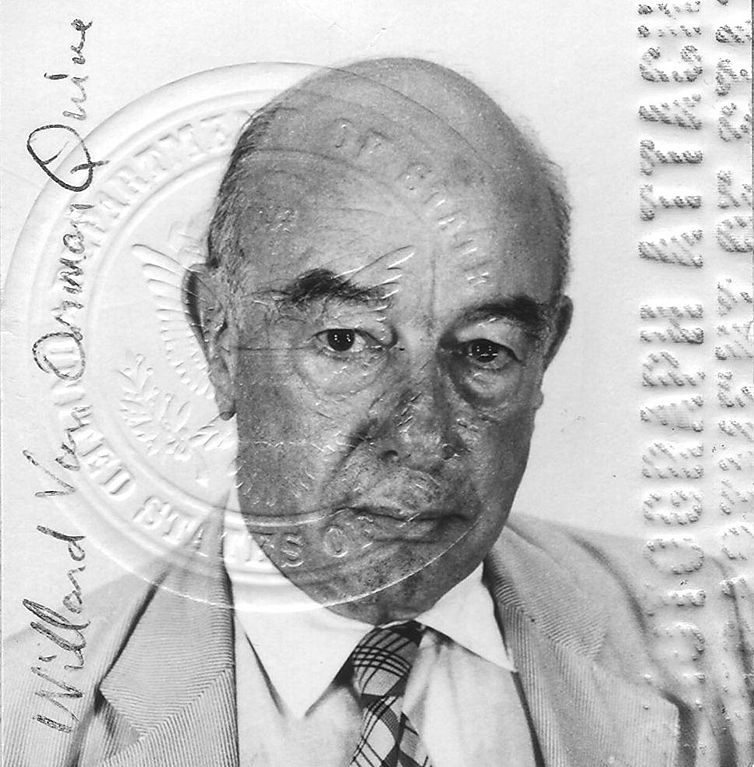# Quine’s Paradox and Gödel’s Theorem

It’s a commonplace to compare Gödel’s theorem to the liar paradox: The sentence

This sentence is not true.

is neither true nor false. Switch out “provable” for “true” and you get

This sentence is not provable.

and, modulo some technical stuff, this sentence is then neither provable nor refutable.  But of course the “modulo some technical stuff” part is crucial: in particular, the Gödel sentence for a theory does not refer to itself. (It does say something about itself, but in a roundabout way.) It can’t refer to itself, because in the theory, you can only refer to sentences via their Gödel numbers, and a sentence can’t contain the numeral for its own Gödel number, very much like a sentence can’t contain itself in quotation marks. But this talk of “the Gödel sentence says of itself that it’s not provable” suggests something like it.  It’s the source of much confusion, and maybe we should avoid the comparison when introducing the incompleteness theorem.

Quine’s Paradox is maybe a bit harder to understand, but it is exactly parallel to the proof of the incompleteness theorem, and in fact the diagonal lemma more generally. Here it is, from The Ways of Paradox:

If, however, in our perversity we are still bent on constructing a sentence that does attribute falsity unequivocally to itself, we can do so thus: ” ‘Yields a falsehood when appended to its own quotation’ yields a falsehood when appended to its own quotation”. This sentence specifies a string of nine words and says of this string that if you put it down twice, with quotation marks around the first of the two occurrences, the result is false. But that result is the very sentence that is doing the telling. The sentence is true if and only if it is false, and we have our antinomy.

Quine’s paradoxical sentence doesn’t refer to itself to produce a paradox.  It just refers to the expression ‘yields a falsehood when appended to its own quotation’, by quotation and then again by the anaphoric ‘it’.  And the Gödel sentence does the same; as Quine puts it:

Gödel’s proof may conveniently be related to the Epimenides paradox or the pseudomenon in the ‘yields a falsehood’ version. For ‘falsehood’ read ‘non-theorem’, thus: ” ‘Yields a non-theorem when appended to its own quotation’ yields a non-theorem when appended to its own quotation”.

This statement no longer presents an antinomy, because it no longer says of itself that it is false. What it does say of itself is that it is not a theorem (of some deductive theory that I have not yet specified). If it is true, here is one truth that that deductive theory, whatever it is, fails to include as a theorem. If the statement is false, it is a theorem, in which event that deductive theory has a false theorem and so is discredited.

What Gödel proceeds to do, in getting his proof of the incompletability of number theory, is the following. He shows how the sort of talk that occurs in the above statement — talk of non-theoremhood and of appending things to quotations — can be mirrored systematically in arithmetical talk of integers. In this way, with much ingenuity, he gets a sentence purely in the arithmetical vocabulary of number theory that inherits that crucial property of being true if and only if not a theorem of number theory. And Gödel’s trick works for any deductive system we may choose as defining ‘theorem of number theory’.

The proof of the diagonal lemma goes something like this. We’re proving that for every $$\psi(x)$$ there is a $$\phi$$ such that $$\phi \leftrightarrow \psi(\ulcorner \phi \urcorner)$$.  Think of $$\psi(x)$$ as naming a kind, the $$\psi$$s, say, falsehoods or non-theorems.  Then to get $$\phi$$ which is true iff it is a $$\psi$$, we proceed as follows: Define the function $$d$$ which maps the Gödel number of $$\alpha(x)$$ to the Gödel number of $$\alpha(\ulcorner \alpha(x)\urcorner)$$.  Supposing we have a function symbol in the language for $$d$$, we can define $$\phi$$ as $$\psi(d(\ulcorner \psi(d(x))\urcorner))$$. If a theory $$T$$ represents $$d$$ then it proves $$d(\ulcorner \psi(d(x))\urcorner) = \ulcorner\phi\urcorner$$. Then, by logic, we also get $$\psi(d(\ulcorner \psi(d(x))\urcorner)) \leftrightarrow \psi(\ulcorner\phi\urcorner)$$. But the left-hand side is just $$\phi$$, so $$T \vdash \phi \leftrightarrow \psi(\ulcorner\phi\urcorner)$$.

Here ‘$$\psi(x)$$’ plays the role of ‘falsehood’, ‘non-theorem’, etc. $$d$$ is the function that takes an expression and appends it to its own quotation.  And $$\phi$$ is $$d$$ applied to ‘yields a $$\psi$$ if appended to its own quotation’, i.e.,

‘yields a $$\psi$$ if appended to its own quotation’ yields a $$\psi$$ if appended to its own quotation.

That sentence does not refer to itself, so it doesn’t say of itself in that sense that it is a $$\psi$$. But it is equivalent to the statement that it is a $$\psi$$.

I don’t know which textbooks, if any, mention Quine’s paradox when introducing the diagonal lemma.  I’m teaching incompleteness right now at McGill from a version of Jeremy Avigad’s notes, where he makes the connection. Let’s see how it goes over in class.

[Photo courtesy of Douglas Quine, via Stampit at Wikimedia Commons licensed under CC-BY-SA-3.0]

## 4 thoughts on “Quine’s Paradox and Gödel’s Theorem”

1.Kayue says:

Maybe you are interested in this paper (if you haven’t read it before): arxiv.org/abs/math/0606425
And Findlay’s one on the reference list.

2.James Walsh says:

Richard Heck’s expository notes on the Diagonal Lemma are in the spirit of Quine’s Paradox:

3.B says: